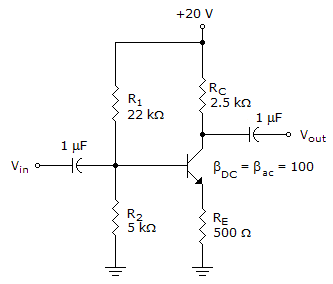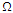# Electronic Devices - BJT Amplifiers - Discussion

### Discussion :: BJT Amplifiers - General Questions (Q.No.21)

21.

Refer to this figure. Calculate the value of Rin(tot).[A]. 37.7 k[B]. 3.77 k[C]. 378[D]. 2.25 kExplanation:

No answer description available for this question.

 K.Rekha said: (Sep 27, 2012) Because range of out put impedance is 40 kilo ohms to 50 kilo ohms. So above options which value is nearer to the resistance that is correct answer.

 Anil From Iiit Basar,Civil said: (Feb 22, 2013) Zi = RB||Zb where Zb = beta.re+(1+beta)RE Zb = (100*4.34)+(1+100)*0.5= 486k ohms Zi = 4.07k || 486k = 3.77k ohms.

 Tuan Tieu said: (Apr 16, 2014) Without bypass capacitor, we have: Rin(base) = beta x (r'e + RE ) = 100 x (6 + 500) = 50k. Rin(total) = R1 || R2 || Rin(base) = 3.767 kOhm.

 Fibo said: (Feb 22, 2017) Where did you get the value of r'e?

 Divya said: (Sep 3, 2017) How to find the RE?

 Charl said: (Sep 29, 2017) re' = 26mV (voltage @ room temp) divided by Ie. Ie = (Vb - Vbe) / RE = (3.7V - 0.7)/500 Get the value of Vb by using voltage divider equation.